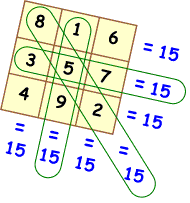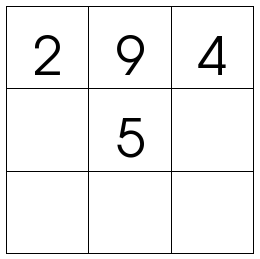# Homework: Compass Directions

Use the compass and the map of Victoria to explain the directions from one place to another.

Horsham to Bendigo is…

Geelong to Bendigo is…

Morwell to Benella is…

Melbourne to Morwell is…

Apollo Bay to Cavendish is…

Your homework is due next Tuesday 8/9/2015

# Maths Rotations: Magic Addition Squares

For maths today we are going to use magic addition squares. A magic addition  square is a square with 3 rows and 3 columns. Each grid of the square has to have a number in it and every line (horizontal, vertical and diagonal needs to add up to the same number – the magic number). Below are two images of a magic addition squares. One of the squares is complete and the other is incomplete. We will work out the incomplete square together.When we complete magic addition squares we need to use our addition strategies to assist us in adding the numbers together.

At the end of the session we will talk about the addition strategies that we were able to use.

# Subtraction Games

Here are some subtraction games that we are going to play today.

This one the kids are given the answer and have to fill in the other two numbers.

http://www.ictgames.com/countonconvict.html

This one uses counting on to the closest ten, then counting the tens then adding the extras. Looks really good!

http://www.abcya.com/math_bingo.htm

Maths Bingo. Have to calculate the answers to the sums and try to get a line.

# Double-Double Strategy When Multiplying By 4

When multiplying by 4 you can use the strategy of double-double. For example, if the problem was 4 x 15, you double 15 (30) and then double 30, which equals 60.

Try solving some of these by using the double-double strategy and post your working out and answers in a comment under this post.

1) 4 x 18
2) 4 x 34
3) 4 x 62
4) 4 x 223

# MATH WORD PROBLEMS

Time to see who is looking at the blog over the long weekend. Here are four word problems that you can have go at working out and solving. You will need to think about what each question is asking, think about how you’re going to solve the problem and please check your answers before posting them as a comment. Please number your answers so they match the questions.

1. A student was working on publishing an information report on their computer. On Monday they typed 237 words. On Tuesday, the student typed 46 more words than they typed on Monday. How many words is their information report?

2. 82 students competed in the 800m race (2 laps around the oval). 13 students stopped during the first lap and 27 students stopped during the second lap. How many students finished the race?

3.  At the beginning of the year each student needed 4 books. If there were 21 students, how many books were needed?

4. Two grades worked on a project together. One grade had 19 students and the other grade had 21. The teachers split them up into 8 equal groups. How many were in each group?